### Algebra Fundamentals

In this quiz we explore various sequences, which not only make for fun puzzles but are central to algebra.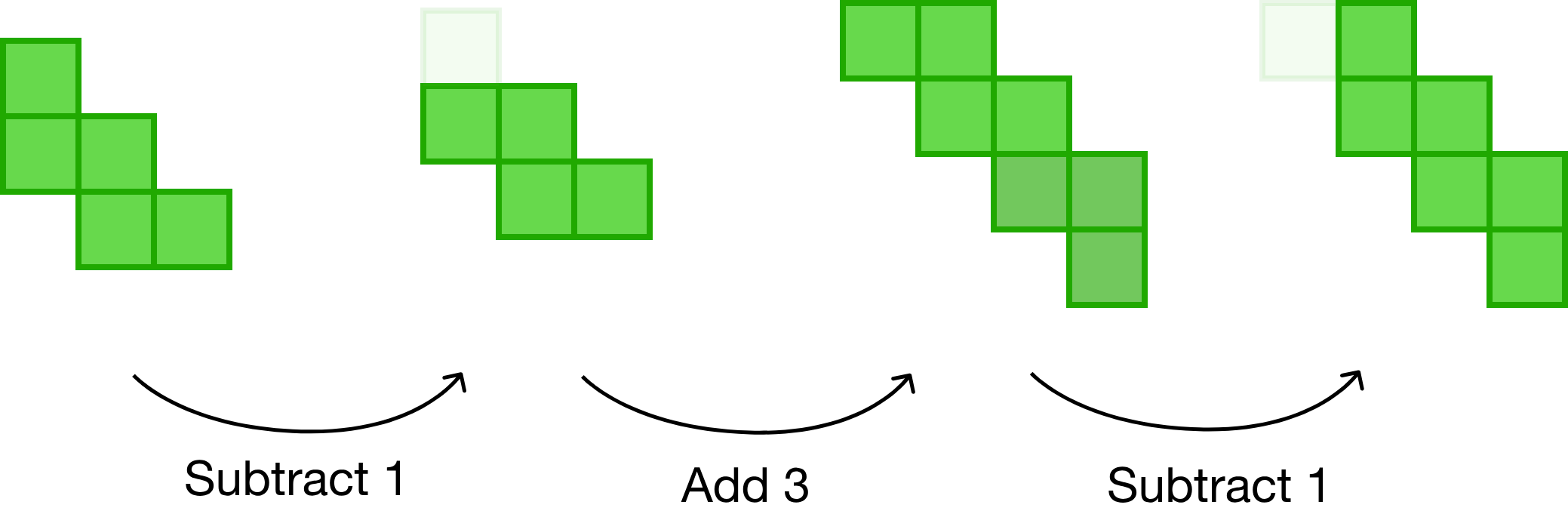Could you describe this pattern well enough that someone could reproduce it without having seen it?

# Sequences

How many squares will be in Step $99?$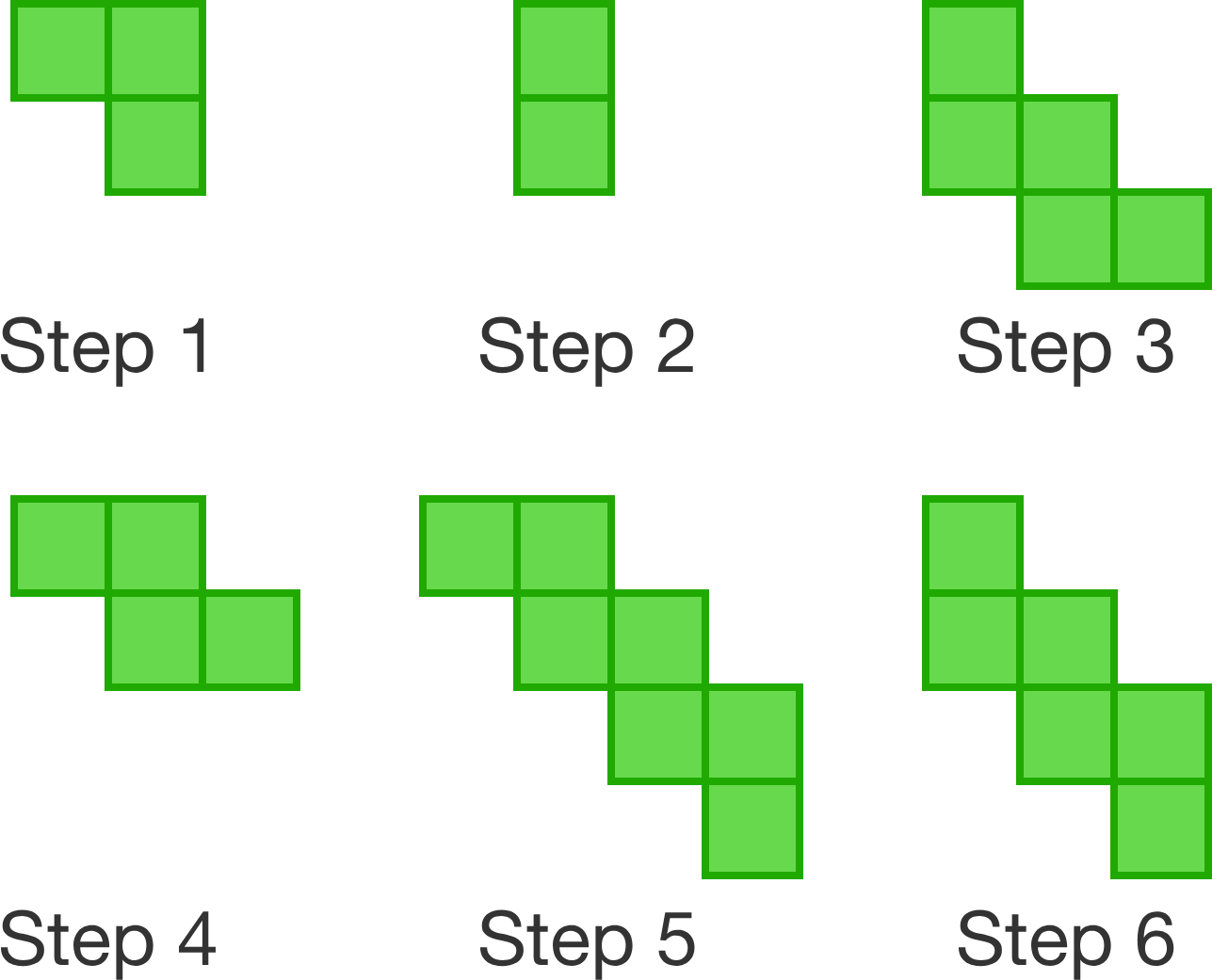# Sequences

Does column $15$ have more stars or squares?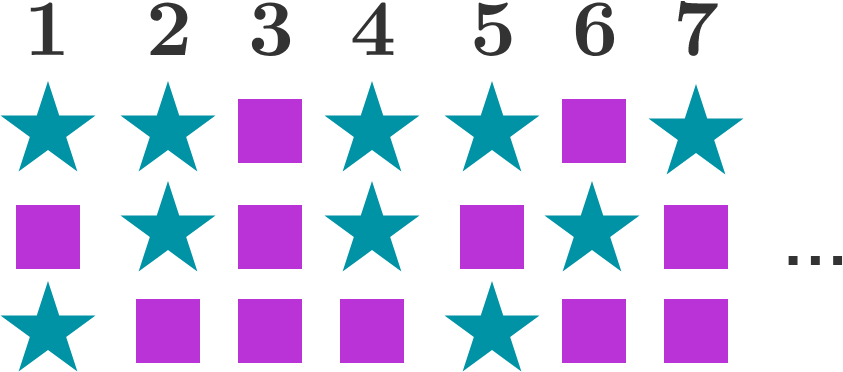Hint: notice that each horizontal row has its own pattern.

# Sequences

In each figure, squares are either empty or filled with a circle. How many empty squares does Figure $10$ have?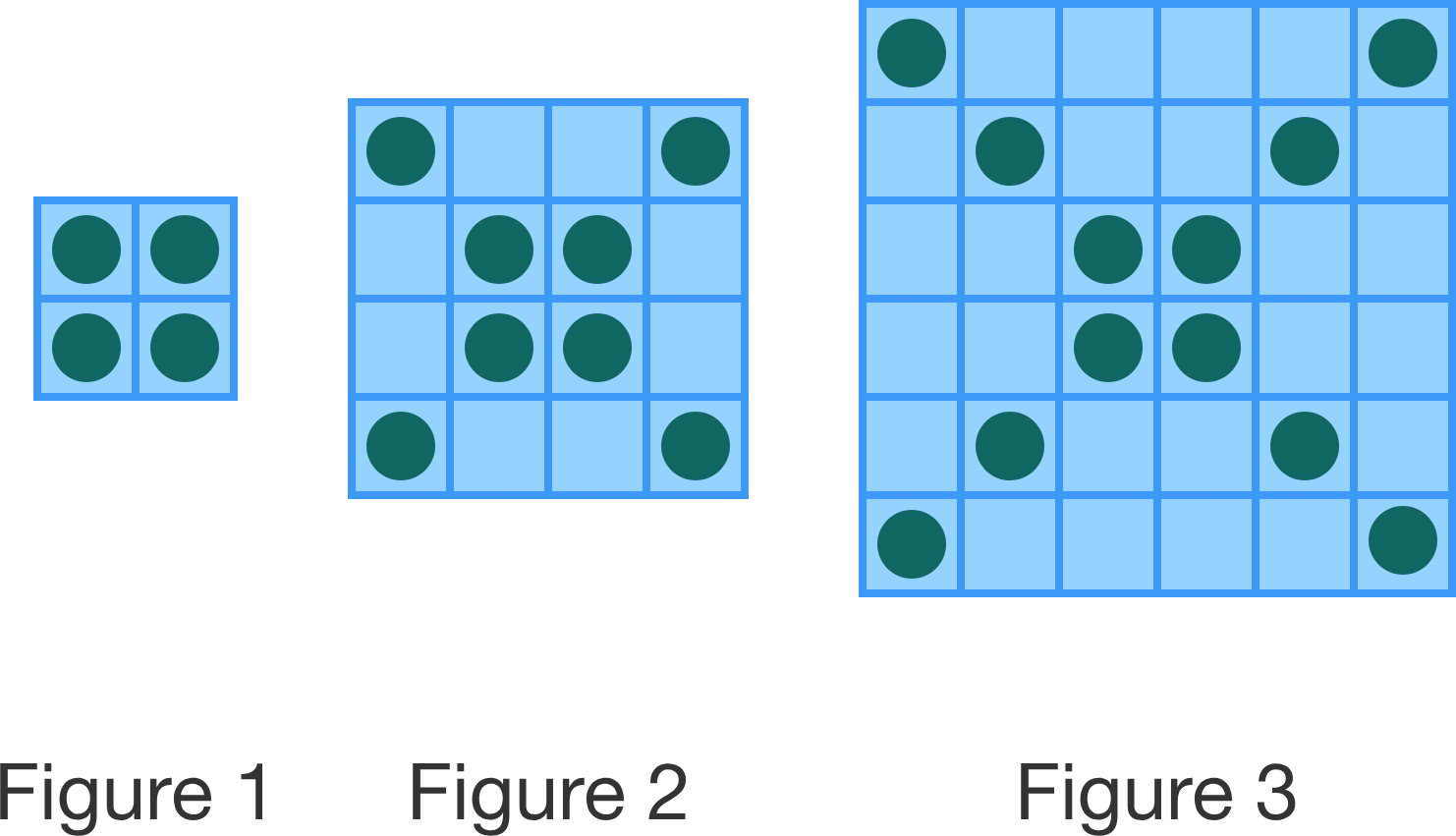# Sequences

$s = 1$ has one unit cube and $s=2$ has $3$ additional unit cubes. Assuming the pattern continues as shown, how many unit cubes will $s = 4$ have?

Note: You can play with the orientation of the blocks.

# Sequences

Extending patterns as we've done in the preceding questions is a foundational algebra skill.

While we can solve many problems like these in a straightforward manner, the use of variables, equations, and algebraic concepts unlocks a variety of additional problems.

# Sequences

Jill is arranging tables for a party that will be placed in one long row, end to end. Each side of each table can seat one person.

Which table shape cannot seat exactly $50$ people, regardless of the number of tables used?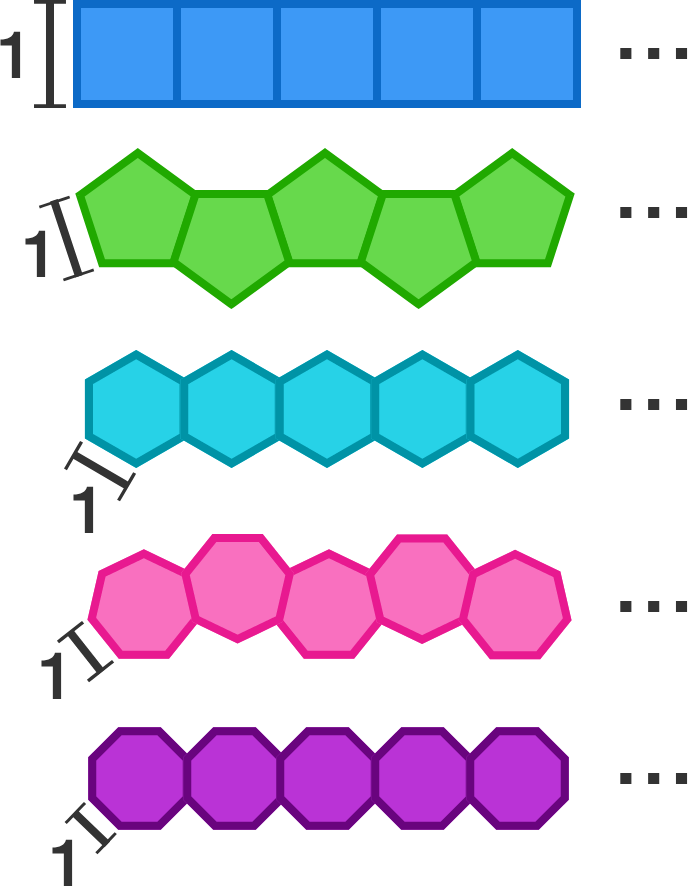×